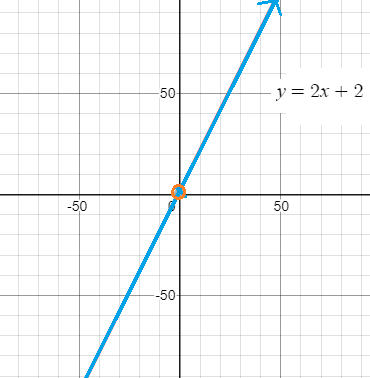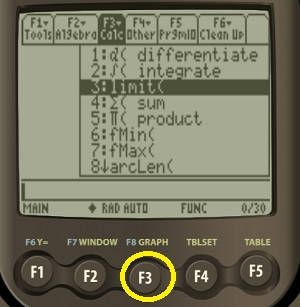# TI-89 Limit in Easy Steps

TI 89 Calculus > TI-89 limit

## TI-89 Limit: OverviewThe limit for this function is 0 at x = 0, and ∞ for x = ∞
A “limit” is the value a function “approaches” as the input or index (usually the x-value) approaches some specified value. In other words, it’s a theoretical point where your function maxes out. Some functions don’t actually reach a certain value; they come close, but not quite.

## Syntax

With its limit command, the TI-89 makes it a snap to evaluate limits. The syntax for the function is:
limit (expression, variable, value).

• “Expression” is just the equation your want to find the limit for.
• “Variable” is nearly always going to be x (although check your equation!)
• “value” is where you want to find the limit.

You can access the limit() function in three different ways (which all access the same command):

• Press F3 (Calc) and then 3 (see worked example below).
• Look in the Catalog (press l to go to the start of the l section).
• Press 2nd, then MATH. Look in the “A:Calculus” submenu.

## TI-89 Limit Example 1

Example problem: Evaluate the limit as x approaches 8 for the following function.
f(x) = (x2 – 4) / (x2 – 6)

Step 1: Press the HOME key.

Step 2: Press the F3 button and then press 3 to select the “limit” command.Step 3: Type your function into the calculator, followed by:
, x , 8 )
We’re typing “x” here and then “8” because that’s where we’re evaluating the limit (at x = 8).Make sure to use parentheses correctly to ensure the right order of operations.

Don’t forget to close your parentheses.

Step 4: Press .
The solution is 15/14.

The nice thing about finding the TI-89 limit is that after you’ve typed in your function and pressed ENTER, it will give you the limit and show you what function you’ve evaluated, so you know you’ve typed in the right formula!

Sometimes your problem might be phrased differently, as in this next example.

## Limit Example 2

Example problem: Describe the limits of the function f(x) = (x3 + 7) / (x2 + 3) symbolically as x approaches 0.

Step 1: Press the HOME key.

Step 2: Press F3 and then 3 to select the limit command.

Step 3: Press ( X ^ 3 + 7 ) ÷ ( X ^ 2 + 3 ) , X , 0

Step 4: Press ENTER.

The solution is 7/3 or 2.3333.

Tips:

1. If you get the error “too few arguments,” make sure you have entered the correct amount of parentheses.
2. The “X” variable and the multiplication symbol “x” look almost identical on the TI-89 keypad. Make sure you are pressing the right key—look to the equation to ensure you have typed the correct function into the calculator.

That’s how to find a TI-89 Limit. You’re done!

References:
TI-89 Simulator (Images).
Clausen, C. Applications of Calculus I: Chemical Kinetics The Derivative as a Function
.
Larson & Edwards. Calculus.

CITE THIS AS:
Stephanie Glen. "TI-89 Limit in Easy Steps" From StatisticsHowTo.com: Elementary Statistics for the rest of us! https://www.statisticshowto.com/ti-89-limit-in-easy-steps/